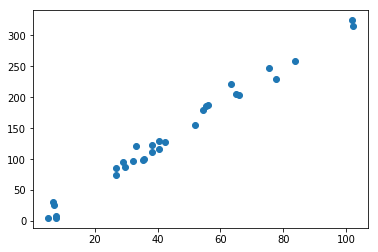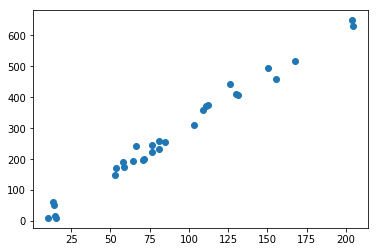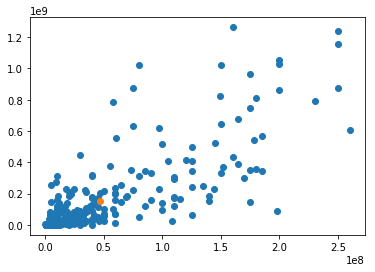## Fitting a linear model to data¶

We started by defining a simple linear model: $Y = mX + b$. It has two variables, the input $X$ and the output $Y$, and two parameters, the slope $m$ and the intercept $b$.

If we had $m$ and $b$, we could make a prediction about $Y$ given any $X$. In this case, we'll assume that we have a lot of examples of pairs of inputs and outputs $X_i, Y_i$. The process of guessing good values of $m$ and $b$ given example inputs and outputs is called linear regression.

A good way to think about $m$ is as the amount you would expect $Y$ to increase if you increase $X$ by 1. The fact that the model is linear means that it doesn't matter what $X$ is, adding 1 to it will always change our guess for $Y$ by $m$.

If the linear model was exactly correct, all of the $X, Y$ pairs would be in a single line. And we could take any two points and find the slope of that line just by dividing the difference in $Y$ values and the difference in $X$ values. But we rarely encounter data like that. There might be errors in our measurements, or additional factors that we don't know about. To simulate this additional randomness, our model is actually $Y = mX + b + error$. The $mX+b$ part is the systematic variability that we are modeling, the $error$ term is everything else.

What makes a good linear model? We want to account for as much variability as we can with the systematic part $mX + b$. For each value of $X$ that we have, our model predicts that the associated $Y$ will be $mX + b$. The error between the actual $Y_i$ and the prediction is called the residual. We want small residuals.

In the previous class we talked about measures of association between two variables, including covariance and correlation coefficient. They both have the same numerator: $\sum_i (X_i - \bar{X})(Y_i - \bar{Y})$. In this class we added one more, an estimate of a slope coefficient $\hat{m}$. It has the same numerator, but it divides that numerator by the variance of $X$.

In :
import numpy
from matplotlib import pyplot
import pandas


Since it's 3/14 $\pi$ day, we'll use linear models to estimate the most famous linear relationship in mathematics: the ratio between the circumference and diameter of a circle.

If $X$ is diameter and $Y$ is circumference, then $Y = \pi X + 0$. The $b$ term is zero because a circle with zero diameter has zero circumference.

Let's create some imaginary "measurements" of circles. If we measure exactly, we would only need two circles to recover $\pi$ exactly, so I'm going to add a bit of error.

In :
## random diameters from 5 to 105
diameters = numpy.random.random(size=30) * 100 + 5
errors = numpy.random.normal(0, 1, size=30)
circumferences = diameters * numpy.pi + 10 * errors

In :
pyplot.scatter(diameters, circumferences)
pyplot.show()It looks like there's a clear linear trend, as expected. Can we measure the strength of the association? Let's look at a covariance matrix. Remember, the diagonal entries [0,0] and [1,1] of this matrix are the variances of the two variables individually, and the off-diagonal entries [1,0] and [0,1] are both the covariance between the variables.

In :
cov_matrix = numpy.cov(diameters, circumferences)
cov_matrix

Out:
array([[ 711.25999118, 2296.83174403],
[2296.83174403, 7517.98676523]])

Our estimate of the slope will then be the covariance divided by the variance of the $diameter$ variable in [0,0].

In :
estimated_pi = cov_matrix[1,0] / cov_matrix[0,0]
estimated_pi

Out:
3.2292435572120435

Pretty close! We didn't get 3.14159 exactly because we added some random error to our circumference measurements.

### What does it mean to divide the covariance by the variance?¶

Covariance measures the association between the variables. If a value $X_i$ is above its mean $\bar{X}$, is the associated $Y_i$ usually above or below $\bar{Y}$? If the product of the centered values $(X_i - \bar{X})(Y_i - \bar{Y})$ tends to be positive, then the plot will look like it's going up, and if that product tends to be negative, the plot will look like it's going down.

So why isn't that enough by itself? Why do we need to divide by the variance? Here's an experiment: Let's multiply all of our circumferences and diameters by 2.

In :
pyplot.scatter(2 * diameters, 2 * circumferences)
pyplot.show()It looks exactly the same; only the axis labels have changed. The relationship between circumference and diameter is no different. But what happens to the variance and covariance?

In :
double_cov_matrix = numpy.cov(2 * diameters, 2 * circumferences)
print("OLD\n", cov_matrix)
print("NEW\n", double_cov_matrix)
print("ratio\n", double_cov_matrix / cov_matrix)

OLD
[[ 711.25999118 2296.83174403]
[2296.83174403 7517.98676523]]
NEW
[[ 2845.03996473  9187.32697611]
[ 9187.32697611 30071.94706091]]
ratio
[[4. 4.]
[4. 4.]]


If we double the values of the diameter and circumference, the variance and covariance increase by a factor of 4! ($2^2$) But because they all change by the same factor, the estimate of the slope (and therefore $\pi$) is unchanged:

In :
double_cov_matrix[1,0] / double_cov_matrix[0,0]

Out:
3.2292435572120435

Let's not forget about the intercept term $b$. This is the value we expect for $Y$ if $X$ is 0. We said it should be 0, but because we are dealing with noisy observations we don't expect it to be exact.

Once we get our estimate of the slope $\hat{m}$ ("m hat"), we can set our estimate of the intercept $\hat{b}$ to the difference between the mean of $Y$ and $\hat{m}$ times the mean of $X$.

In :
numpy.mean(circumferences) - estimated_pi * numpy.mean(diameters)

Out:
-5.005295415392055

## Linear models on movies¶

I chose the $\pi$ example because it's definitely a linear relationship. But in the real world relationships between variables are rarely exactly linear. As with the "spot the Poisson" exercise, our goal is to decide whether a linear model is "good enough".

In :
movies = pandas.read_csv("movies.csv")

In :
pyplot.scatter(movies.budget, movies.revenue)
pyplot.scatter(movies.budget.mean(), movies.revenue.mean())
pyplot.show()This isn't nearly as clear as the $\pi$ example. The points look much more dispersed, and not all in a line. But there are some suggestive "gaps". If we look at the four quadrants around the orange mean point, the top-left "low budget/high revenue" quadrant (indie hits like Clerks, Slumdog Millionaire) is pretty empty. The bottom-right "high budget/low revenue" (massive flops like John Carter, Fantastic Four) is also sparse.

Let's look at the covariance matrix.

In :
movie_cov = numpy.cov(movies.budget, movies.revenue)
movie_cov

Out:
array([[3.18938774e+15, 1.07111151e+16],
[1.07111151e+16, 5.79288099e+16]])

These numbers are huge! But that's mostly because the underlying numbers are really big. If we normalize by the variance of the budget, we get an estimate of a slope of 3.36. That means that if we believe this is a good model, a movie that spends an extra dollar should make an extra \$3.36. In : movie_slope = movie_cov[1,0] / movie_cov[0,0] movie_slope  Out: 3.3583609082992893 We can also calculate the slope going the other way: a movie with one dollar of additional revenue would be expected to cost \$.18 more. That's because the variance of revenue is so much bigger than budget.

In :
movie_cov[1,0] / movie_cov[1,1]

Out:
0.18490134932687835

Finally, let's calculate the intercept: the amount of money a movie with a budget of \$0 should expect to make. In : numpy.mean(movies.revenue) - movie_slope * numpy.mean(movies.budget)  Out: -5341441.999101102 This shows where the linear model starts to break down. I can't lose \$5M on a movie I spent nothing on.

If you were a studio exec, would you use this model to decide how much to spend on your next movie? Most of you thought no. But you were also pretty convinced that higher budgets are associated with (but don't necessarily cause) higher revenue.